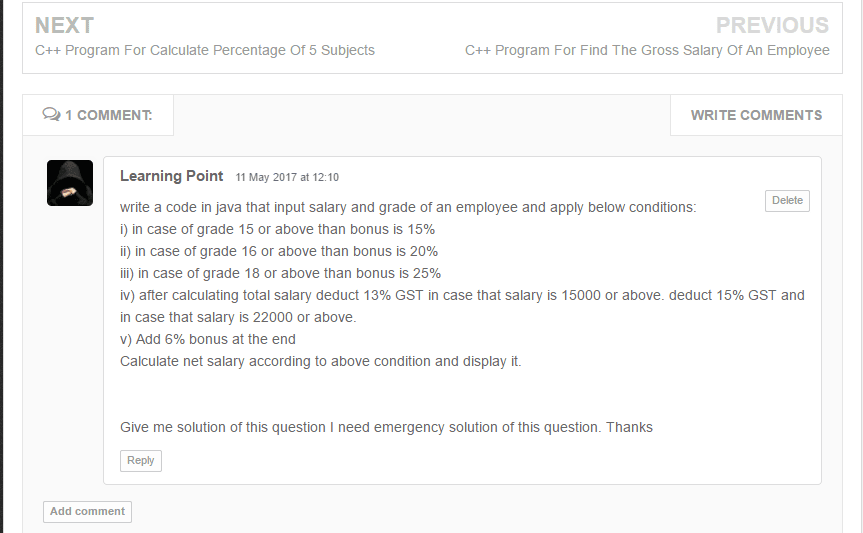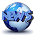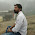# Java Program to Find Salary of an Employee With Grade

Problem:- Java program for salary of an employee or java program for find the salary of an employee with employee grade or simple employee program in java or employee details program in java or employee salary calculation program in java or employee details program in java using inheritance or java program for employee details using array or java program for employee payroll or employee salary calculation program in java,

Note:- Please see this is a requested problem from Pakistan. See you can also request any problem regarding programming. So don't forget to share thanks, learning point (chowk Azam, Pakistan) writing for us.

Write a code in java that input salary and grade of an employee and apply conditions
1. in case of grade 15 or above than bonus is 15%
2. in case of grade 16 or above than bonus is 20%
3. in case of grade 18 or above than bonus is 25%
4. after calculating total salary deduct 13% GST in case that salary is 15000 or above. Deduct 15% GST and in case that salary is 22000 or above.
5. add 6% bonus at the end
Calculate net salary according to above condition and display it.Logic:- so according to first three conditions in the problem I use if-else statement for a grade of employee see below.

```if(grade==15)
{
bonus=(basic_salary*15)/100;
}
{
bonus=(basic_salary*20)/100;
}
{
bonus=(basic_salary*25)/100;
}```

After that, we get a basic salary + bonus. Now according to GST( Goods and Service Tax ) conditions calculate a remaining salary after all calculation 6% bonus.

```if(basic_salary>=15000 && basic_salary<22000 basic_salary-="gst;" basic_salary="" bonus1="" else="" gst="(basic_salary*13)/100;" if="">=22000)
{
gst=(basic_salary*15)/100;

basic_salary-=gst;

bonus1=(basic_salary*6)/100;

basic_salary+=bonus1;

}
else
{
bonus1=(basic_salary*6)/100;

basic_salary+=bonus1;
}```

At the end print the Salary.

Example:- let's assume employee salary is 20,000 and grade are 15 then calculate an employee salary.

20,000 salary and 15 grade bonus is 15% then bonus = 3000.

• Now total salary is 20,000+3000=23,000
• Now salary is greater than 22,000 then 15% GST, so GST = 3450.
• Now total salary is 23,000 - 3450 = 19550.
• At the end 6% bonus then bonus = 1173
• so gross salary of an employee is =19500+1173=20723.

Gross salary = 20723.

Try This Java Program To Find The Gross Salary Of An Employee

### Solution:- Java Program for Find The Salary of an Employee With Employee Grade

import java.util.Scanner;
import java.lang.*;

/* Program By Ghanendra Yadav
Visit http://www.programmingwithbasics.com
*/

class employeesalary
{
public static void main(String args[])
{
float basic_salary,bonus1=0,bonus=0,gst;

Scanner scan=new Scanner(System.in);

System.out.println("Enter Basic Salary Of Employee : ");
basic_salary=scan.nextFloat();

System.out.println("\nEnter The Grade Of Employee : ");

{
bonus=(basic_salary*15)/100;
{
bonus=(basic_salary*20)/100;
}
{
bonus=(basic_salary*25)/100;
}

basic_salary+=bonus;

if(basic_salary>=15000 && basic_salary=22000)
{
gst=(basic_salary*15)/100;

basic_salary-=gst;

bonus1=(basic_salary*6)/100;

basic_salary+=bonus1;
}
else
{
bonus1=(basic_salary*6)/100;

basic_salary+=bonus1;
System.out.println("\nGross Salary Of Employee : "+basic_salary);
}
}

Output:-

1.1.2.